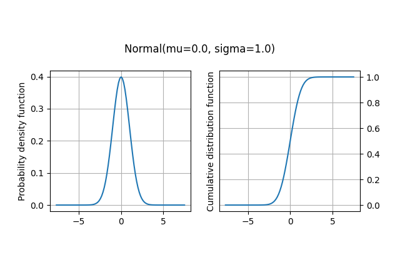gemseo / uncertainty / distributions / openturns

# normal module¶

Class to create a normal distribution from the OpenTURNS library.

This class inherits from OTDistribution.

class gemseo.uncertainty.distributions.openturns.normal.OTNormalDistribution(variable='x', mu=0.0, sigma=1.0, dimension=1, transformation=None, lower_bound=None, upper_bound=None, threshold=0.5)[source]

Bases: OTDistribution

Create a normal distribution.

Examples

>>> from gemseo.uncertainty.distributions.openturns.normal import (
...     OTNormalDistribution
>>> )
>>> distribution = OTNormalDistribution('x', -1, 2)
>>> print(distribution)
Normal(mu=-1, sigma=2)

Parameters:
• variable (str) –

The name of the random variable.

By default it is set to “x”.

• mu (float) –

The mean of the normal random variable.

By default it is set to 0.0.

• sigma (float) –

The standard deviation of the normal random variable.

By default it is set to 1.0.

• dimension (int) –

The dimension of the random variable. If greater than 1, the probability distribution is applied to all components of the random variable under the hypothesis that these components are stochastically independent. To be removed in a future version; use a ComposedDistribution instead.

By default it is set to 1.

• transformation (str | None) – A transformation applied to the random variable, e.g. $$\sin(x)$$. If None, no transformation.

• lower_bound (float | None) – A lower bound to truncate the probability distribution. If None, no lower truncation.

• upper_bound (float | None) – An upper bound to truncate the probability distribution. If None, no upper truncation.

• threshold (float) –

A threshold in [0,1].

By default it is set to 0.5.

dimension: int

The number of dimensions of the random variable.

distribution: ot.ComposedDistribution

The probability distribution of the random variable.

distribution_name: str

The name of the probability distribution.

marginals: list[ot.Distribution]

The marginal distributions of the components of the random variable.

math_lower_bound: ndarray

The mathematical lower bound of the random variable.

math_upper_bound: ndarray

The mathematical upper bound of the random variable.

num_lower_bound: ndarray

The numerical lower bound of the random variable.

num_upper_bound: ndarray

The numerical upper bound of the random variable.

parameters: tuple[Any] | dict[str, Any]

The parameters of the probability distribution.

standard_parameters: dict[str, str] | None

The standard representation of the parameters of the distribution, used for its string representation.

transformation: str

The transformation applied to the random variable, e.g. ‘sin(x)’.

variable_name: str

The name of the random variable.

## Examples using OTNormalDistribution¶Probability distributions based on OpenTURNS

Probability distributions based on OpenTURNS